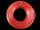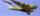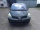# Unit conversion + functions - math problems

#### Number of problems found: 5

• Electrics - conductorThe wire is 106 meters long at 0 °C and at every temperature increase of 1 °C the length increases by 0.15 mm per 1 m length of wire. Determine a function which represents the overall length of the wire as a function of temperature. What is the length of
• AircraftThe aircraft has in a fuel tank 68 hl of aviation fuel and flight consumes 3.6 liters of fuel. Identify the function, which expresses the dependence of the volume of fuel in tank on the track distance plane flew by. How many hectolites of fuel is still in
• TrigonometryIf you know that cos(γ) = sin (806°), what is the angle γ?
• Used carsPeter plans to buy a used car: the first car Renault Espace 2.0 dCi 16V Dynamique 2006, costs 2000 euros. It is 14 years old and has a combined diesel consumption of 8 liters. / 100 km. Diesel costs 1.1 euros/liter. How much will the car cost him to opera
• Degrees Fahrenheit? The equation above shows how temperature F, measured in degrees Fahrenheit, relates to a temperature C, measured in degrees Celsius. Based on the equation, which of the following must be true? I. A temperature increase of 1 degree Fahrenheit is equivale

We apologize, but in this category are not a lot of examples.
Do you have an interesting mathematical word problem that you can't solve it? Submit a math problem, and we can try to solve it.

We will send a solution to your e-mail address. Solved examples are also published here. Please enter the e-mail correctly and check whether you don't have a full mailbox.

Please do not submit problems from current active competitions such as Mathematical Olympiad, correspondence seminars etc...